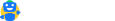# Dividend Yield Ratio: Calculation, Formula, Analysis, Explanation

Updated on:

The dividend yield is the amount a company pays to its investors as dividends in comparison with the current market price of the stock.

## What is Dividend Yield Ratio?

The dividend yield is a way to measure how much cash flow you are getting for each rupee invested in an equity position. In the absence of any capital gains, dividends are treated as the return on stock investment.

Investors can be classified as growth-oriented and value-oriented investors, where the former invests in growing companies and expects returns in the form of capital gains as the company grows over time. Value-oriented investors, on the other hand, expect stable returns in the form of dividends along with capital gains over the long term. The dividend yield ratio is highly useful for value-oriented investors.

## Formula of Dividend Yield Ratio

The dividend yield ratio is calculated using the following formula:

Dividend Yield Ratio = Dividend Per Share/Market Value Per Share.

In the simplest form of calculation, you can take the amount of dividend per share and divide it with the market value per share to get the dividend yield ratio. However, companies tend to announce the dividends as gross dividends distributed.

In that case, it will have to be divided by the outstanding common stock in that year. The market value of the share is taken as the one at the end of the tenure in question.

## How to Calculate Dividend Yield Ratio?

Say a company has announced Rs 10,00,000 as cash dividend to be paid during the current year. The number of outstanding common stock is 10,000. So, the dividend that each share will earn would be as follows:

Dividend per share = Total cash dividend/Outstanding Common Stock

= 10,00,000/10,000 = 100

So, the dividend per share would be Rs 100. To calculate the dividend yield ratio, we will have to divide this by the current market price. If the current market price of the share is Rs 1,000, we get the following:

## Analysis

As we saw earlier, dividends are essential for investors who wish to make a continuous passive income from their investments. The dividend yield ratio will give you the productivity of your investments. Dividends are also considered as a sign of a stable company since only profit-making companies pay dividends.

Historically, companies that pay dividends mostly continue to do so as a dividend cut is received adversely by the markets. The companies that offer regular dividends are considered as mature companies since it shows the control over liquidity position. Since value investors prefer this, such companies do well even in the time of market lows.

## Explanation

Dividend yield ratio is one of the several parameters that are used to evaluate a company before purchasing their stocks. Investors also use forward dividend yield ratio and trailing dividend yield ratio to get a better understanding of the company’s dividend yield. The dividend yield ratio is significantly different across several industries. Industries such as IT or electronics are known to have a negligible dividend yield.

On the contrary, PSU or FMCG can have a stable dividend yield. It is essential to understand that not all companies with a high dividend yield ratio are worth investing. If the market price of the share is falling, the dividend yield ratio becomes more attractive. In such an instance, the company might not be a good buy.

Finally, it is also vital to understand that the stability in dividend yield and strong fundamentals are a few of the positive indicators for purchasing a stock. One should also consider other macroeconomic factors such as government policies before investing.Invest in Direct Mutual Funds
Save taxes upto Rs 46,800, 0% commissionHave a query?
mailto@cleartax.in
Company
Individuals
Tax Experts
SMEs
Enterprises
ITR Resources
MF Resources
Tools
ClearGST
ClearServices